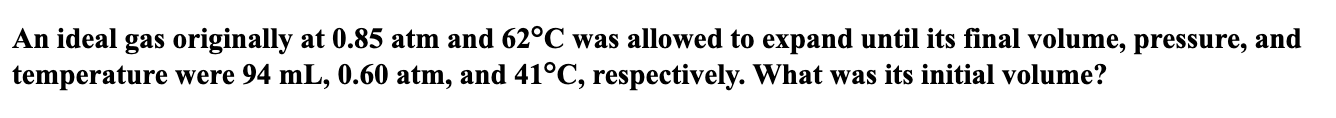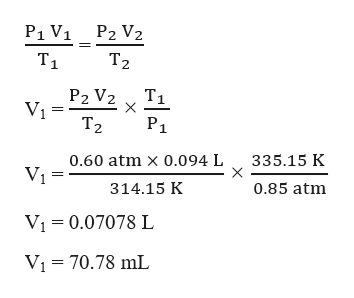An ideal gas originally at 0.85 atm and 62°C was allowed to expand until its final volume, pressure, andtemperature were 94 mL, 0.60 atm, and 41°C, respectively. What was its initial volume?

Questionhelp_outlineImage TranscriptioncloseAn ideal gas originally at 0.85 atm and 62°C was allowed to expand until its final volume, pressure, and temperature were 94 mL, 0.60 atm, and 41°C, respectively. What was its initial volume? fullscreen
Step 1

Given,

Initial pressure, P1 = 0.85 atm

Initial pressure, T1 = 62 oC = (62 + 273.15) K = 335.15 K

Initial pressure, V1 = ?

Final pressure, P2 = 0.60 atm

Final pressure, T2 = 41 oC = (41 + 273.15) K = 314.15 K

Final pressure, V2 = 94 mL = 0.094 L

Step 2

The initial volume of the ideal ...help_outlineImage TranscriptioncloseP1 V1 P2 V2 T1 Тz T1 P2 V2 Тz Р1 0.60 atm x 0.094 L 335.15 K 314.15 K 0.85 atm Vi 0.07078 L VI 70.78 mL fullscreen

Want to see the full answer?

See Solution

Want to see this answer and more?

Our solutions are written by experts, many with advanced degrees, and available 24/7

See Solution
Tagged in

Chemistry